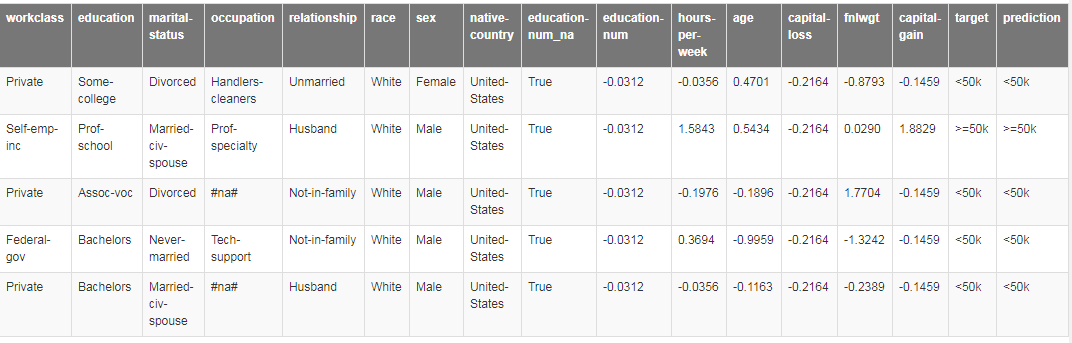# fastai 官方教程之查看数据

### 总目录：

• 查看数据：本节为初级教程，介绍怎样快速的查看你的数据和模型预测结果。

• 推理学习器（Inference Learner）：本节为中级教程，介绍怎样为（模型）推理创建学习器。

• 自定义类ItemList（Custom ItemList）：本节为高级教程，介绍如何创建类ItemBase与类ItemList。

• 使用极小的代价实现深度学习：本节介绍如何使用较少的GPU资源实现推理，和避免重启笔记内核的时间。

### 查看数据

• 查看输入与输出
• 视觉任务
• 分类问题
• 多标签问题
• 回归任务示例
• 分割任务示例
• 文本任务
• 语言模型
• 文本分类
• 列表（Tabular）

#### 视觉

from fastai.vision import *


mnist = untar_data(URLs.MNIST_TINY)tfms = get_transforms(do_flip=False)


data = (ImageList.from_folder(mnist)
.split_by_folder()
.label_from_folder()
.transform(tfms, size=32)
.databunch()
.normalize(imagenet_stats))


data.show_batch()data.show_batch(rows=3, figsize=(4,4))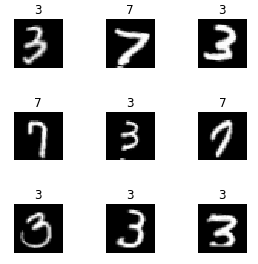learn = cnn_learner(data, models.resnet18, metrics=accuracy)learn.fit_one_cycle(1,1e-2)
learn.save('mini_train')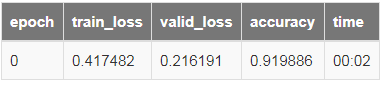learn.show_results()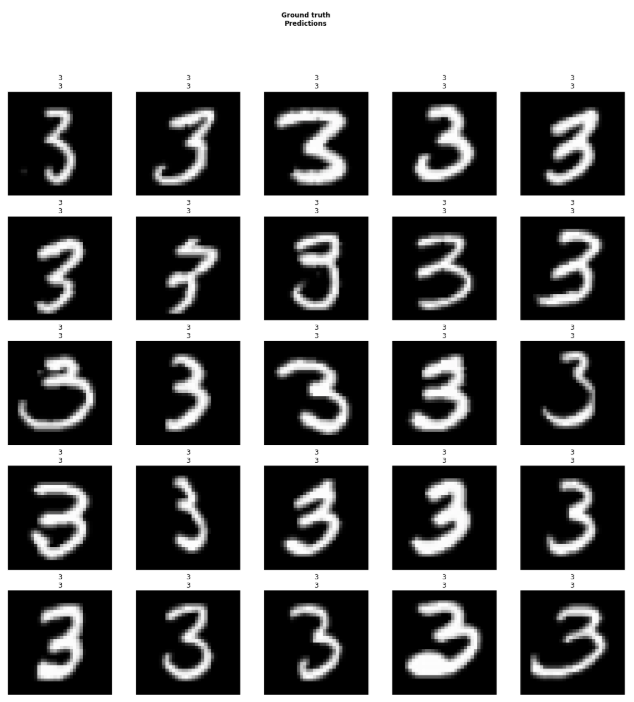learn.show_results(ds_type=DatasetType.Train, rows=4, figsize=(8,10))planet = untar_data(URLs.PLANET_TINY)
planet_tfms = get_transforms(flip_vert=True, max_lighting=0.1, max_zoom=1.05, max_warp=0.)


data = (ImageList.from_csv(planet, 'labels.csv', folder='train', suffix='.jpg')
.split_by_rand_pct()
.label_from_df(label_delim=' ')
.transform(planet_tfms, size=128)
.databunch()
.normalize(imagenet_stats))


data.show_batch(rows=2, figsize=(9,7))learn = cnn_learner(data, models.resnet18)
learn.fit_one_cycle(5,1e-2)
learn.save('mini_train')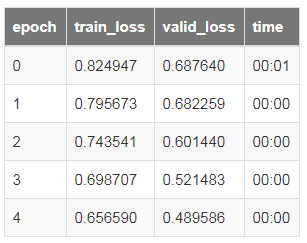learn.show_results(rows=3, figsize=(12,15))biwi = untar_data(URLs.BIWI_SAMPLE)


data = (PointsItemList.from_folder(biwi)
.split_by_rand_pct(seed=42)
.label_from_func(lambda o:fn2ctr[o.name])
.transform(get_transforms(), tfm_y=True, size=(120,160))
.databunch()
.normalize(imagenet_stats))


data.show_batch(row=3, figsize=(9,6))learn = cnn_learner(data, models.resnet18, lin_ftrs=, ps=0.05)
learn.fit_one_cycle(5, 5e-2)
learn.save('mini_train')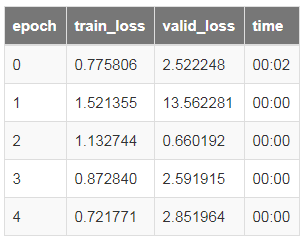learn.show_results(rows=3)camvid = untar_data(URLs.CAMVID_TINY)
path_lbl = camvid/'labels'
path_img = camvid/'images'


codes = np.loadtxt(camvid/'codes.txt', dtype=str)
get_y_fn = lambda x: path_lbl/f'{x.stem}_P{x.suffix}'


data = (SegmentationItemList.from_folder(path_img)
.split_by_rand_pct()
.label_from_func(get_y_fn, classes=codes)
.transform(get_transforms(), tfm_y=True, size=128)
.databunch(bs=16, path=camvid)
.normalize(imagenet_stats))

data.show_batch(rows=2, figsize=(7,5))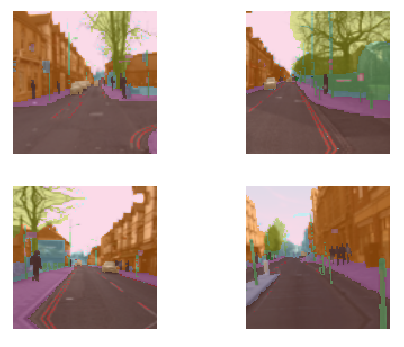learn = unet_learner(data, models.resnet18)learn.fit_one_cycle(3,1e-2)learn.save('mini_train')learn.show_results()#### 文本

from fastai.text import *


imdb = untar_data(URLs.IMDB_SAMPLE)

data_lm = (TextList.from_csv(imdb, 'texts.csv', cols='text')
.split_by_rand_pct()
.label_for_lm()
.databunch())data_lm.save()


data_lm.show_batch()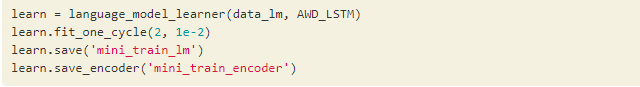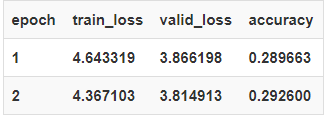learn.show_results()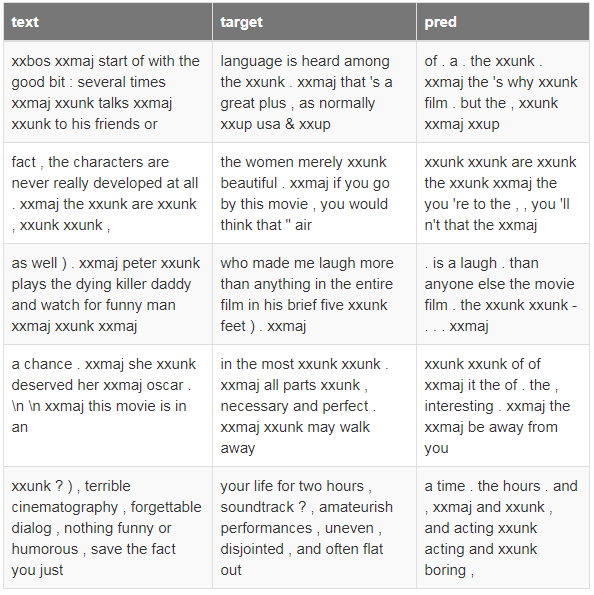data_clas = (TextList.from_csv(imdb, 'texts.csv', cols='text', vocab=data_lm.vocab)
.split_from_df(col='is_valid')
.label_from_df(cols='label')
.databunch(bs=42))


data_clas.show_batch()learn = text_classifier_learner(data_clas, AWD_LSTM)
learn.fit_one_cycle(2, slice(1e-3,1e-2))learn.save('mini_train_clas')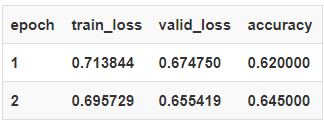learn.show_results()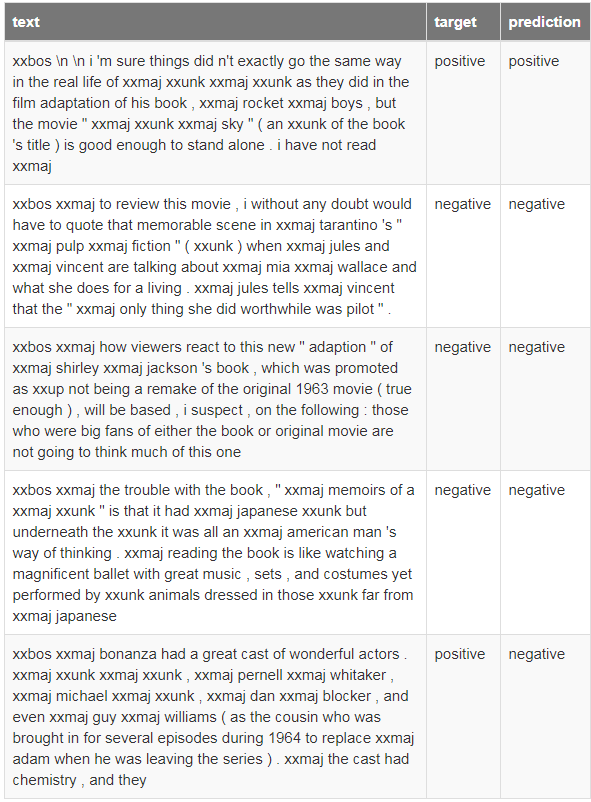#### 列表

from fastai.tabular import *


adult = untar_data(URLs.ADULT_SAMPLE)df = pd.read_csv(adult/'adult.csv')
dep_var = 'salary'
cat_names = ['workclass', 'education', 'marital-status', 'occupation', 'relationship', 'race', 'sex', 'native-country']
cont_names = ['education-num', 'hours-per-week', 'age', 'capital-loss', 'fnlwgt', 'capital-gain']
procs = [FillMissing, Categorify, Normalize]


data = (TabularList.from_df(df, path=adult, cat_names=cat_names, cont_names=cont_names, procs=procs)
.split_by_idx(valid_idx=range(800,1000))
.label_from_df(cols=dep_var)
.databunch())
data.show_batch()learn = tabular_learner(data, layers=[200,100], metrics=accuracy)
learn.fit(5, 1e-2)
learn.save('mini_train')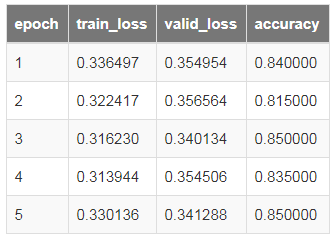learn.show_results()